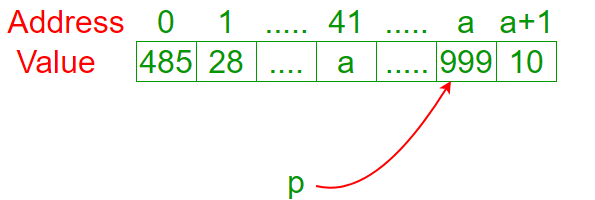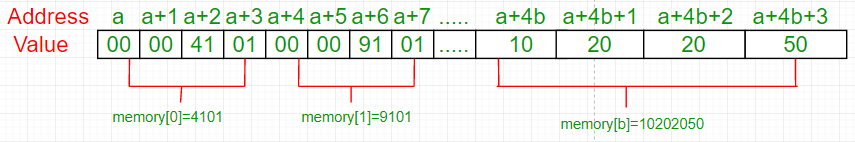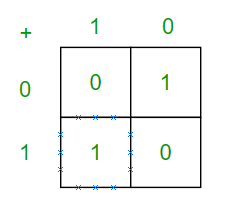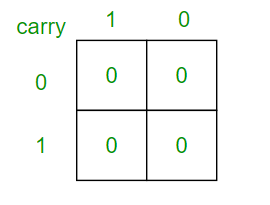Open in App
Not now

# How to sum two integers without using arithmetic operators in C/C++?

• Difficulty Level : Medium
• Last Updated : 12 Jul, 2022

Given two integers a and b, how can we evaluate the sum a + b without using operators such as +, -, ++, –, …?

Method 1 (Using pointers)

An interesting way would be:

## CPP

 `// May not work with C++ compilers and``// may produce warnings in C.` `// Returns sum of 'a' and 'b'``int` `sum(``int` `a, ``int` `b)``{``    ``char` `*p = a;``    ``return` `(``int``)&p[b];``}`

Despite its awkwardness at first sight, we can easily understand what is happening. First, we created a pointer p. The value of a pointer is a memory address. In this case, the value of p is the address a.Remember: p points to position a. In that example, if we want to know the value of position a (999) we ask to *p. If we want to know the address of variable a (41), we ask to &a. If we evaluated p[b], we’d get the value of memory in position p + b. In fact, we evaluate &p[b], which is the same as getting address p + b without accessing its value. As p = a, &p[b] will return the address a + b. We don’t want to return a memory address (int*). We want to return an integer (int). So, we cast &p[b] to int. What happens if we change the type of p from char* to int*? Let me fix what I said before: When we evaluate p[b] we don’t evaluate p + b. We evaluate p + sizeof(*p) * b. Why? Imagine this example: Variables of type int occupies fours positions in the memory.This sizeof(*p) considers the quantity of positions each variable occupies in the memory. We want to evaluate p + b. In other words, we want sizeof(*p) equals to 1. Consequently, if *p is a char, we’re happy.

Method 2 (Using bitwise operators)

Let’s look the sum’s truth table (forgive the carry for now): Looking carefully, we notice that the sum’s and xor’s truth table are the same. It’s 1 only when the input differs. Now, how can we detect carry? Let’s look the carry’s truth table.Looking carefully, we notice that the sum’s and xor’s truth table are the same. It’s 1 only when the input differs. Now, how can we detect carry? Let’s look the carry’s truth table.Looking carefully one more time, we notice that the carry’s and logical and’s truth table are identicals. Now, we have to shift a & 1 to left and sum with a ^ b. However, these operations may have carries as well. No problem, just sum a ^ b with a & 1 shifted to left recursively.

## CPP

 `// Returns sum of a and b using bitwise``// operators.``int` `sum(``int` `a, ``int` `b)``{``    ``int` `s = a ^ b;``    ``int` `carry = a & b;` `    ``if` `(carry == 0) ``return` `s;``    ``else` `return` `sum(s, carry << 1);``}`

This solution has been discussed here.

Method 3 (Using printf)

Let’s remember some facts:

• The printf returns the number of characters printed successfully.
• The specifier %*c requests two parameters: The first one is the customized width and the second is character. For example, printf(“%*c”, 5, ‘a’) will print ” a”.
• The special character ‘\r’ returns the cursor from the beginning of output string. For example, printf(“abcd\r12”) will print “12cd”.

Keeping that in mind, we can understand this function:

## CPP

 `// Returns sum of a and b using printf``// Constraints: a, b > 0.``int` `sum(``int` `a, ``int` `b)``{``    ``return` `printf``("%*c%*c", a, ``'\r'``, b, ``'\r'``);``}`